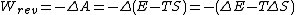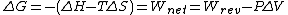# free energy

Also found in: Dictionary, Thesaurus, Medical, Legal, Wikipedia.

## free energy

free energy or Gibbs free energy, quantity derived from the relationships between heat and work studied in thermodynamics and used as a measure of the relative stability of a physical or chemical system, i.e., the tendency of the system to react or change. If the change in free energy, ΔG, is negative, the transformation of the system will occur spontaneously, since transitions in which the energy decreases are favored, whereas those in which it increases (ΔG positive) are not. The change in free energy for a given process at a particular temperature depends on three factors, as seen from the equation ΔG = ΔHTΔS, where ΔH is the change in the enthalpy of the system, T is the temperature in degrees Kelvin, and ΔS is the change in entropy. A negative value of the enthalpy change indicates a decrease in the heat content of the system and contributes to a favorable value of the free energy; a positive entropy change indicates a decrease in the orderliness of the system and also contributes to a favorable value of the free energy, since a system tends to go from more ordered to less ordered states. It may happen that the change in enthalpy for the reaction is favorable but that of the entropy is unfavorable, or vice versa; in such a case the temperature is the deciding factor since it determines how much weight is given to the entropy change. For example, in the transition of liquid water to ice, the enthalpy change is favorable because heat is released in the process but the entropy change is unfavorable because the transition is to the more ordered, crystalline state. Below a temperature of 32℉ (273K) the enthalpy term, ΔH, is larger and the process is spontaneous, but at higher temperatures the entropy term, TΔS, predominates, and the transition does not occur. Although the free energy indicates whether or not a given reaction will occur, it gives no information about the speed of such a reaction. The reaction of hydrogen with oxygen to form water has a favorable, negative, free energy, but the reaction rate is so slow that without the presence of a catalyst it is not observable. Scientists use tables listing the standard free energy, ΔG°, of various compounds; the standard free energy is the change in free energy when one mole of the compound is formed at 25℃ and 1 atmospheric pressure.

## Free energy

A term in thermodynamics which in different treatments may designate either of two functions defined in terms of the internal energy E or enthalpy H, and the temperature-entropy product TS.

The function (ETS) is the Helmholtz free energy and is the function ordinarily meant by free energy in European references. The Gibbs free energy is the function (HTS). For the Lewis and Randall school of American chemical thermodynamics, this is the function meant by the free energy F. To avoid confusion with the symbol F as applied elsewhere to the Helmholtz free energy, the symbol G has also been used. Another development was the introduction of the name free enthalpy, with symbol G, for the Gibbs function. See Work function (thermodynamics)

For a closed system (no transfer of matter across its boundaries), the work which can be done

(1)in a reversible isothermal process is given by the series shown in Eq. (1). For these conditions, TΔS represents the heat given up to the surroundings. Should the process be exothermal, TΔS < 0, then actual work done on the surroundings is less than the decrease in the internal energy of the system. The quantity (ΔE - TΔS) can then be thought of as a change in free energy, that is, as that part of the internal energy change which can be converted into work under the specified conditions. This then is the origin of the name free energy. Such an interpretation of thermodynamic quantities can be misleading, however; for the case in which TΔS is positive, Eq. (1) shows that the decrease in “free” energy is greater than the decrease in internal energy.

For constant temperature and pressure in a reversible process the decrease in the Gibbs function G for the system again corresponds to a free-energy change in the above sense, since it is equal to the work which can be done by the closed system other than that associated with its change in volume ΔV under the given constant pressure P. The relations shown in Eq. (2) can be formed since ΔH = ΔE + PΔV.

(2)Each of these free-energy functions is an extensive property of the state of the thermodynamic system. For a specified change in state, both ΔA and ΔG are independent of the path by which the change is accomplished. Only changes in these functions can be measured, not values for a single state.

The thermodynamic criteria for reversibility, irreversibility, and equilibrium for processes in closed systems at constant temperature and pressure are expressed naturally in terms of the function G. For any infinitesimal process at constant temperature and pressure, -dG ≥ δwnet. If δwnet is never negative, that is, if the surroundings do no net work on the system, then the change dG must be negative or zero. For a reversible differential process, -dG > δwnet; for an irreversible process, -dG > δwnet. The free energy G thus decreases to a minimum value characteristic of the equilibrium state at the given temperature and pressure. At equilibrium, dG = 0 for any differential process taking place, for example, an infinitesimal change in the degree of completion of a chemical reaction. A parallel role is played by the work function A for conditions of constant temperature and volume. Because temperature and pressure constitute more convenient working variables than temperature and volume, it is the Gibbs free energy which is the more commonly used in thermodynamics. See Entropy, Thermodynamic principles

McGraw-Hill Concise Encyclopedia of Physics. © 2002 by The McGraw-Hill Companies, Inc.
The following article is from The Great Soviet Encyclopedia (1979). It might be outdated or ideologically biased.

## Free Energy

a term used to refer to the Gibbs function and to the Helmholtz function, both of which are thermodynamic potentials. In Soviet usage, the term “free energy” is used primarily for the Helmholtz function.

The Helmholtz free energy, also called the work function, is defined as the difference between the internal energy U of a thermodynamic system and the product of the system’s entropy S and temperature T. The quantity ST, which is subtracted from the internal energy when the free energy is found, is sometimes called the bound energy.

## free energy

[¦frē ′en·ər·jē]
(thermodynamics)
The internal energy of a system minus the product of its temperature and its entropy. Also known as Helmholtz free energy; Helmholtz function; Helmholtz potential; thermodynamic potential at constant volume; work function.
McGraw-Hill Dictionary of Scientific & Technical Terms, 6E, Copyright © 2003 by The McGraw-Hill Companies, Inc.
References in periodicals archive ?
After obtaining analytical expressions for the determinants of the matrices of the free energy derivatives of the system under consideration, the positions of the zero contours of the considered derivatives were calculated.
A traditional and common method for determining the disperse and polar (acid-base) components of the surface free energy is a graphical method, which is based on the equation by Owens-Wendt (5) .
The polar and dispersive components of the surface free energy can be calculated using the Young equation, which relates the surface free energy and liquid-solid contact angle and is expressed as follows :
The Young-Dupre equation allows for predictability of adhesion based upon the surface free energy of the liquid in question and the contact angle of the liquid upon the surface in question.
RNA sequences with known secondar structures to get an alternative set of free energy change parameter values.
Conference giving information about free energy. November 20.
Mardan -- Residents stressed for measures to make sure fair and transparent distribution of free energy savers among consumers under the Prime Minister Energy Savers program as launched few days ago in Mardan city like other parts of the country.
RAWALPINDI -- Islamabad Electricity Supply Company (IESCO) has started door to door program of distributing free energy savers (CFL) to the citizens under Energy Conservation Program.
To study problems of the canonical ensemble, we focus on the calculation the canonical density matrix and the Helmholtz free energy of a single particle under asymmetric harmonic oscillator potential.
However, the progress in the development of optical processing systems is being impeded by the lack of enough knowledge on instabilities of FLCs, which is related with the free energy. One of the main advantages in the application of the methods of bifurcation theory to nonlinear stability problems is that in general it is possible to reduce the dimension of the original system to a low dimensional bifurcation system, mainly in the neighborhood of the critical points.

Site: Follow: Share:
Open / Close